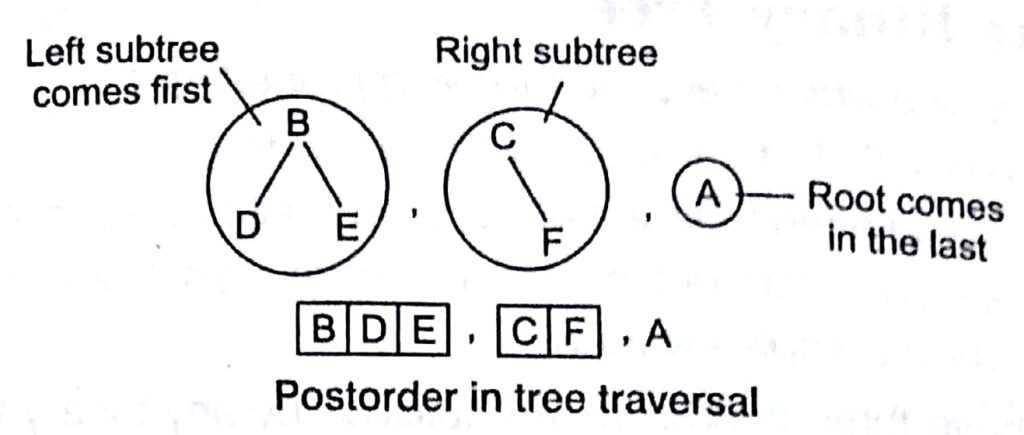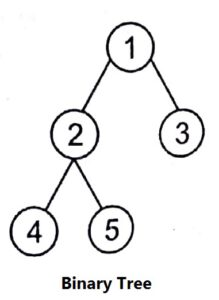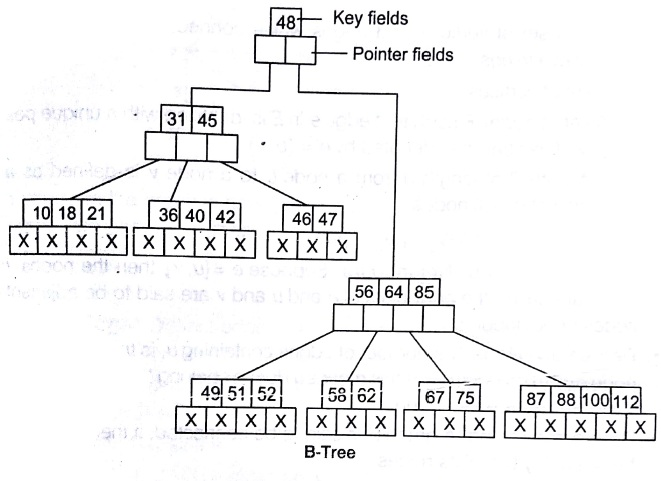# Tree in C Language Tutorial Notes Study Material with Examples

## Tree (Non-linear Data Structures)

Trees are used to represent data containing a hierarchical relationship between elements e.g., records, family trees and table contents

• Node:  Each data item in a tree.
• Root:  First or top data item in hierarchical arrangement.
• Degree of a Node:  Number of subtrees of a given node.

e.g., Degree of A = 3, Degree of E = 2

• Degree of a Tree Maximum degree of a node in a tree.

e.g., Degree of above tree = 3

• Depth or Height Maximum level number of a node + 1(i.e., level number

of farthest leaf node of a tree + 1).

e.g, Depth of above tree = 3 + 1= 4

• Non-terminal Node Any node except root node whose degree is not zero.
• Terminal Node or Leaf Nodes having degree = 0
• Forest Set of disjoint trees.
• Siblings D and G are siblings of parent Node
• Path Sequence of consecutive edges from the source node to the destination node.

e.g. , Path between A and K comprises (A, E), (E, and K) node pairs

## Binary Tree

In this kind of tree, the maximum degree of any node is at most 2. A binary tree T is defined as a finite set of elements such that

• T is empty (called NULL tree or empty tree).
• T contains a distinguished Node R called the root of T and the remaining nodes of T form an ordered pair of disjoint binary trees T1 and T2.
•Any no N in a binary tree T has either 0, 1 or 2 successors. Level r of a binary tree T can have at most 21 nodes.

Extended Binary Trees : 2-Trees or Strictly

## Binary Trees

If every non-terminal node in a binary tree consist of non-empty left subtree and right subtree. In other words, if any node of a binary tree has either 0o2 child nodes, then such tree is known as strictly binary tree or extended

### binary tree or 2- tree.### Complete Binary Tree

A binary tree is one which have the following properties

• Which can have 0, 1 or 2 nodes as a child node.
• In which first, we need to fill left node, then right node in a level.
• In which, we can start putting data item in next level only when the previous level is completely filled. If all the above three conditions are fulfilled by any binary tree, then we can say that as a complete binary tree.
•Now, if we want to add new item in above tree, then we need to add it as right child of node 6. We can’t add it anywhere else. (According to condition 2 and 3).

## Tree Traversal

#### Three types of tree traversal are given below

1. Preorder (Root, Left subtree, Right subtree)
2. Postorder (Left subtree, Right subtree, Root)
3. Inorder (Left subtree, Root, Right subtree)

### Preorder### Postorder### InorderNote: In all of the above three techniques, we need to decompose left and right subtree, according to respective rules only.

The BFT of a tree visits all the nodes in the order of their depth in the tree BFT first visits all the nodes at depth zero (i.e., root), then all the nodes a depth 1 and so on. At each depth, the nodes are visited from left to right.

## Depth First Traversal (DFT)

In DFT, one starts from root and explores as far as possible along eacr branch before backtracking.

### Perfect Binary Tree or Full Binary Tree

A binary tree in which all leaves are at the same level or at the same depth and in which every parent has 2 children. Here, all leaves (D, E, F, G) are at depth 3 or level 2 and every parent is having exactly 2 children.

### Key Points

• BFT uses queue for traversing.
• DFT uses stack for traversing.

### Some Important Formulas of Binary Tree

Number of nodes ‘n’ in a perfect binary tree can be found using the following formula

n =2h1

Where, h= Height of perfect binary tree i.e., number of levels in tree or (maximum level number + 1). The number of nodes ‘n’ in a complete binary tree is at-least (2h -1) and at most (2h — 1).

In a complete binary tree, if we want to find out children and parent of any node k, use following formulaLeft child of the node k =2 * k

Right child of the node k = (2 * k) + 1

Parent of the node k = [k /2]

Left child of node 2 = 2 * 2 = 4

Right child of node 2 = (2 * 2) + 1= 5, here, k = 2

Parent of node 2 = [2/2] =1

The depth dn of the complete binary tree Tn with n nodes is given by

dn=[log2 n +1]

Suppose, any complete tree T, has 8 nodes, then

dn =[ log2 8 +1.]= [ log223 +1] = [3 log22 + 1 ]

dn = [ 3+1 ]=4

The number of nodes in a perfect binary tree can also be found using formula n =2L – 1,where L is the number of leaf nodes in the trees number of null links in a binary tree of  ‘n’  nodes is equal to (n + 1).

## Binary Search Tree (BST)

A binary tree T, is called binary search tree (or binary sorted tree), if each node N of T has the following property. The value at N is greater than ever, value in the left subtree of N and is less than or equal to every value in the  right subtree of N.

## AVL-Tree

The disadvantage of a BST is that if every item which is inserted to be next is greater than the previous item, then we will get a right skewed BST or if every item which is to be inserted is less than to the previous item, then we will get a left skewed BST.

so, to overcome the skewness problem in BST, the concept of AVL-tree height balanced tree came into existence.

A non-empty binary tree T is an AVL-tree if and only if

h (TL     ) — h (T) |  ≤ 1

where, h ((TL     ) = Height of left subtree TL of tree T

h (TR) = Height of right subtree TR of tree T

h (TL  ) — h (TR) is also known as Balance Factor (BF). For an AVL (or height balanced tree), the balance factor can be either 0,1 or —1. An AVL search tree is binary search tree which is an AVL-tree.h(TL. ofC)= 1

h (TR of C)=2

BF of               C = h(TL of C) — h (TR of C) C = 1 — 2

C = —1

### Key Points

• In the AVL-tree, every node should have the value of BF either 0 or 1 or —1.
• If the value of BF is not falling in this criteria, then that tree is not an AVL-tree.

## m-way Search Tree

To favour retrieval and manipulation of data stored in external memory viz. storage devices such as disks etc., there is a need for some special data structures. e.g., such data structures as m-way search trees. B-Trees and B+-trees. An m-way search tree T may be an empty tree, if T is non-empty, it satisfies the following properties

• For some integer m, known as order of the tree, each node is of degree which can reach a maximum of m, in other words, each node has at most m child nodes.
• A node may be represented as Ao, (k1, A1), (k2, A2), … (km _ 1. Am1), where k;,1 i (m — 1) are the keys and A, 0 < i < (m – 1) are the pointers to subtrees of T.
• If a node can have k child nodes, where k < m, then the node can have and each of the keys partitions all the keys in the subtrees into k subsets.i + 1 only (k — 1) keys k1, k2,…kk _ 1, contained in the node such that ki  < ki  + 1 and each of the keys partitions all the keys in the subtrees into k subsets.
• For a node Ao, (ki, A1), (k2, A2),…, (km.1, Am _ 1), all key value in the subtree ointed to by A1 are less than the key k,,i 0 < i < m -2 and all key values in the subtree pointed to by km_ 1 are greater than Km-1.
• Each of the subtrees A1, 0 ≤ I ≤ m– 1 are also m—way search trees.
• Below is an example of a 5-way search tree. Here, m=5 which each node can have at most m= 5 child nodes and therefore has almost (m –1= 4) keys contained in it.
•In the above diagram, we can see that k1 < k2 < k3 < k4 for each node.

## B-Tree

m-way search trees have the advantage of minimizing file accesses due to their restricted height. But still, we need to keep the height of m-way search tree as low as possible and therefore the need to maintain balanced m-way search trees arises.

So, a B-tree is nothing but a balance m-way search tree. A B-tree of order m, if non-empty, is an m-way search tree in which

• The root has at-least  2 child nodes and at most m child nodes.
• The internal nodes except the root have at-least [m/2] child nodes and at most m child nodes.
• The number of keys in each internal node is one less than the number of child nodes and these keys partition the keys in the subtrees of the node in a manner similar to that of m-way search trees.
• All Leaf nodes are on the same level.
• A B-tree of order 5 is referred to as 4 – 5 tree’, since the internal nodes  are of degree 2 or 3 only.
•## B+ Trees

• It is a variant of B-tree in which all records are stored in the leaves and all leaves are linked sequentially.
• A B+ tree consists of one or more blocks of data, called nodes linked together by pointers.
• In a B+-tree, in contrast to a B -tree, all records are stored at the lowest level of the tree.
• Internal nodes point  to other  nodes in the tree  and leaf nodes point to data in database using  data pointers.